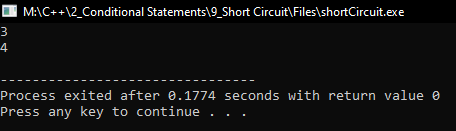# Short Circuit in C++

## Short Circuit in C++ with Examples:

In this article, I am going to discuss Short Circuit in C++ with Examples. Please read our previous article where we discussed Else If Ladder in C++ with Examples.

##### Short Circuit in C++

Let us see what is a short circuit.

If (a > b && a > c)

Here, we have a condition which is ‘a’ greater than ‘b’ and ‘a’ greater than ‘c’, and we used the logical ‘AND’ – “&&” operator. This ‘&&’ will be true if both ‘a>b’ and ‘a>c’ are true. If anyone is false then the &&’ will be false. Suppose ‘a>b’ is false means ‘a’ is not greater than ‘b’. So, if ‘a>b’ is false then the next condition will not be checked i.e. ‘a>c’ will not be checked. So, this is called short circuit.

In the same way, if we write, If (a > b || a > c)

Here we are using logical ‘OR’ – “||” Operator. If any one of the conditions is true then ‘||’ will be true. If ‘a>b’ is true then ‘a>c’ will not be checked because one condition should be true that is sufficient. This mechanism is done by the compiler is Short Circuit.

Let see another example, if we have 3 variables,

int a = 5, b = 7, i = 5;

And we write a condition as,

If (a > b && ++i < b)

cout << i ;

Here first we are checking for ‘a>b’ means 5 is greater than 7? No, so ‘a>b’ is false and here we are performing ‘&&’, so if any condition fails in AND then the entire ‘&&’ will be false. So, if ‘a>b’ is false then it will not check for ‘++i < b’ because already the first condition is false. After that if we print the value of ‘i’ then it will not be incremented, it will remain unchanged as ‘5’.

Note: In the second part of the conditional statement, never use increment or decrement operators because they may be or may not be executed.

##### Program for Short Circuit in C++:
```#include <iostream>
using namespace std;
int main()
{
int a = 10, b = 5, i = 3, j = 4;

if (a < b && ++i <= b)
{
// no code here
}
cout << i << endl;

if (a > b || ++j <= b)
{
cout << j << endl;
}

return 0;
}
```
###### Output:Note: It is the optimization technique compiler follows when they are evaluating logical operators. C++ short circuit happens for &&, || operators.

In the next article, I am going to discuss Dynamic Declaration in C++ with Examples. Here, in this article, I try to explain Short Circuit in C++ with Examples and I hope you enjoy this Short Circuit in C++ with Examples article.